# Data formatting guide: Tudor

• Updated

## Summary

Learn about the process of formatting an Opening Balance and General Ledger file from Tudor.

## Configuration details

This guide is broken into 2 parts:

A raw Excel export from Tudor will look like the image below.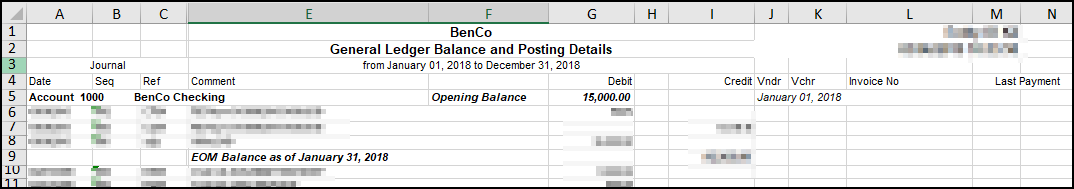## Part 1: Format an opening balance

1. Begin by filtering the “opening balance” in the column containing the opening balance (column F in this example).2. Copy and paste the filtered opening balances into a new workbook and save it as a new file for import.3. Consolidate debit and credit values into a single column called “Balance” by using the formula below. Debits will be positive, credits will be negative. =IF(ISNUMBER(I2),I2,K2*-1)
Note: This formula searches for an available debit value. If a debit value is present, it will be used. If no debit value is found, then the credit value is multiplied by the negative one to create a negative value.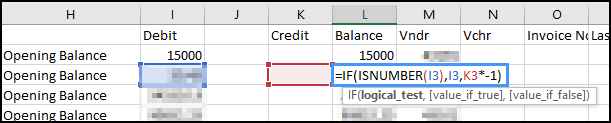4. Copy and paste the values for a new “Balance” column.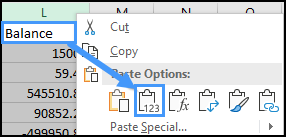5. Remove all columns except "Account Number", "Account Description" and "Balance."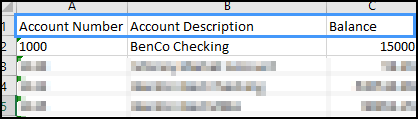The same steps can be taken to format the closing balance file.

## Part 2: Format the general ledger

1. Save a copy of the General Ledger (GL) file as a .csv.2. Re-open the newly saved .csv file in Excel, which is now formatted as shown in the image below.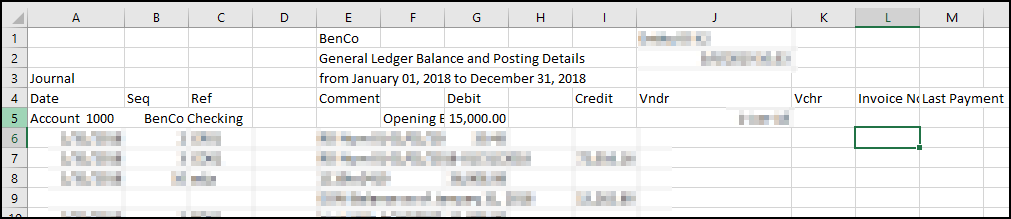3. Remove all rows above the “date” cell.4. Create 2 new columns named "Account Number" and "Account Description."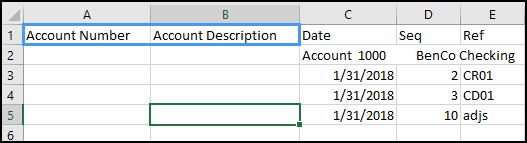5. Copy the account number by using the following formula: =IF(IFERROR(SEARCH("account",C2),FALSE), MID(C2,10,4),A1)
Note: this formula is searches for the text “account” in column C.
• If “account” is found, then the formula will take the four digit account number by counting 10 characters into the cell, then taking the next four digits.
• If “account” is not found, the formula copies the cell above in the B column. This process allows for the formula to be copied down the entire GL.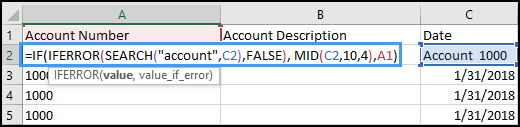6. Copy the account description using the following formula: =IF(IFERROR(SEARCH("account",C2),FALSE), MID(C2,24,100),B1)
Note: This formula uses the logic applied above, but instead captures the account description.7. Copy both formulas to the bottom of the worksheet, then copy and paste each column as values to remove the formulas.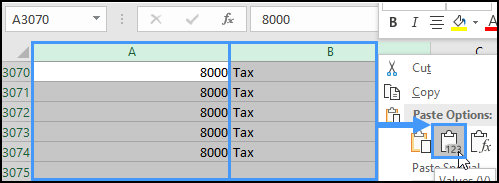8. Remove unnecessary rows of data by filtering the “Date” column.
9. Using the filter, deselect the date values: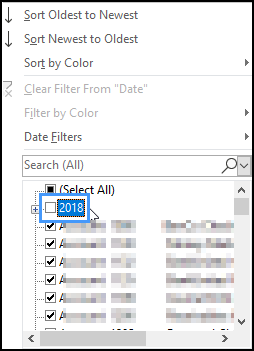10. Delete the filtered rows: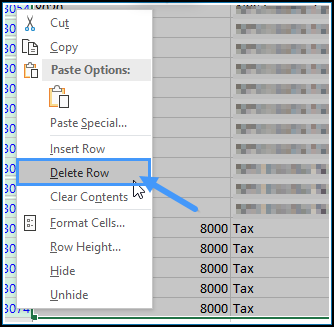11. Remove blank columns and rename remaining the following columns:
Column New Column Name
Date Posted Date
Comment Memo
12. Your final data file will look like the image below.